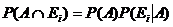# Statistics Assignment Help With Bayes Theorem

## 2.9 Bayes Theorem:

If E1, E1,………. En are mutually disjoint events with P(Ei) ≠0, (i= 1, 2,…….,n) then for any arbitrary event A which is a subset of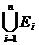such that P(A) >0 we have, i =1, 2,…n

Proof:Since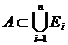, we have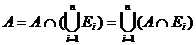Since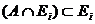(i=1,2…..n) are mutually disjoint events, we have by addition theorem of probability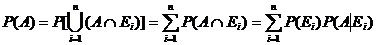By compounded theorem of probability.

Also we have